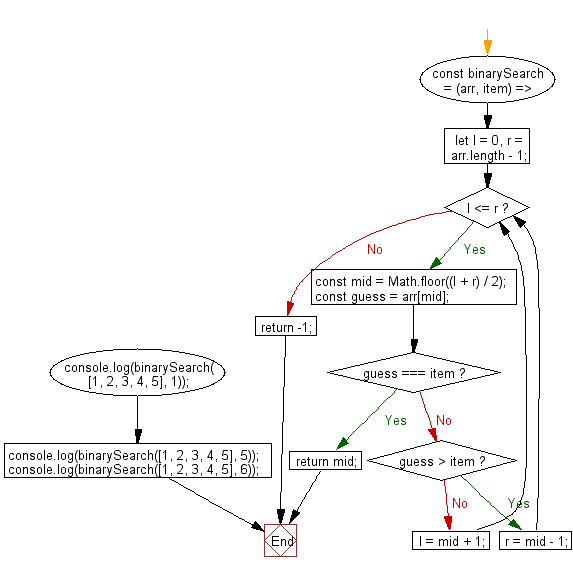# JavaScript Sorting Algorithm: Find the index of a given element in a sorted array using the binary search algorithm

## JavaScript Sorting Algorithm: Exercise-21 with Solution

Write a JavaScript program to find the index of a given element in a sorted array using the binary search algorithm.

• Declare the left and right search boundaries, l and r, initialized to 0 and the length of the array respectively.
• Use a while loop to repeatedly narrow down the search subarray, using Math.floor() to cut it in half.
• Return the index of the element if found, otherwise return -1.
• Note: Does not account for duplicate values in the array.

Sample Solution:

JavaScript Code:

``````const binarySearch = (arr, item) => {
let l = 0,
r = arr.length - 1;
while (l <= r) {
const mid = Math.floor((l + r) / 2);
const guess = arr[mid];
if (guess === item) return mid;
if (guess > item) r = mid - 1;
else l = mid + 1;
}
return -1;
};

console.log(binarySearch([1, 2, 3, 4, 5], 1));
console.log(binarySearch([1, 2, 3, 4, 5], 5));
console.log(binarySearch([1, 2, 3, 4, 5], 6));
```
```

Sample Output:

```0
4
-1
```

Flowchart:Live Demo:

See the Pen javascript-common-editor by w3resource (@w3resource) on CodePen.

Improve this sample solution and post your code through Disqus

What is the difficulty level of this exercise?

Test your Programming skills with w3resource's quiz.

﻿